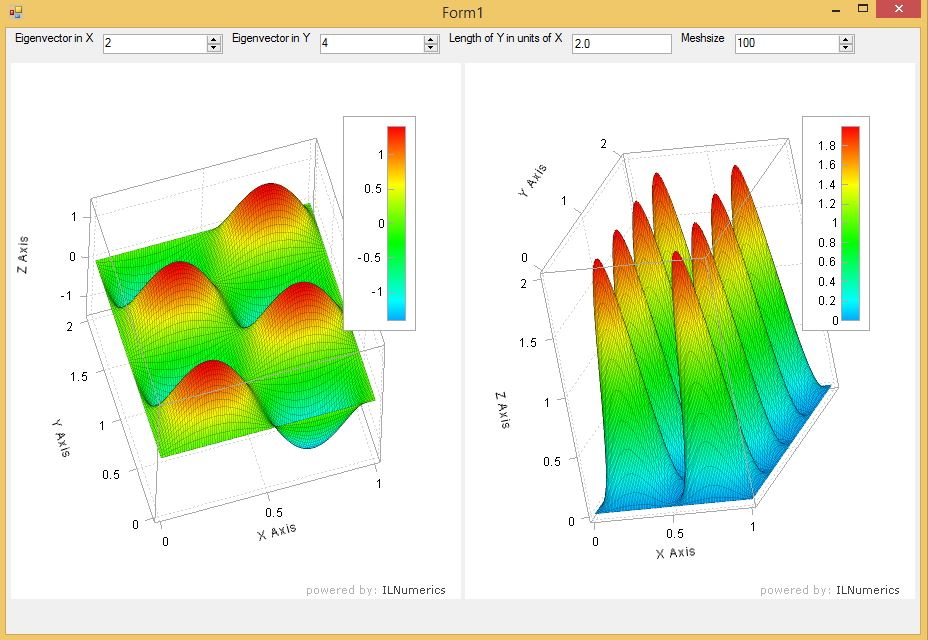# ILNumerics for Scientists – Going 3D

### Recap

Last time I started with one of the easiest problems in quantum mechanics: the particle in a box. This time I’ll add 1 dimension and we’ll see a particle in a 2D box. To visualize its wave function and density we need 3D surface plots.

### 2D Box

This time we have a particle that is confined in a 2D box. The potential within the box is zero and outside the box infinity. Again the solution is well-known and can be found on Wikipedia. This time the state of the wave function is determined by two numbers. These are typically called quantum numbers and refer to the X and the Y direction, respectively.

The absolute size of the box doesn’t really matter and we didn’t worry about it in the 1D case. However, the relative size of the length and the width make a difference. The solution to our problem reads

$\Psi_{n,k}(x,y) = \sqrt{\frac{4}{L_x L_y}} \cdot \sin(n \cdot \pi \cdot x / L_x) \cdot \sin(k \cdot \pi \cdot y / L_y)$

### The Math

Very similar to the 1D case I quickly coded the wave function and the density for further plotting. I had to make sure that the arrays are fit for 3D plotting, so the code looks a little bit different compared to last post’s

     public static ILArray<double> CalcWF(int EVXID, int EVYID, double LX, double LY, int MeshSize)
{
ILArray<double> X = linspace<double>(0, LX, MeshSize);
ILArray<double> Y = linspace<double>(0, LY, MeshSize);

ILArray<double> Y2d = 1;
ILArray<double> X2d = meshgrid(X, Y, Y2d);

ILArray<double> Z = sqrt(4.0 / LX / LY) * sin(EVXID * pi * X2d / LX) * sin(EVYID * pi * Y2d / LY);

return Z.Concat(X2d,2).Concat(Y2d,2);
}


Again, this took me like 10 minutes and I was done.

### The Visualization

This time the user can choose the quantum numbers for X and Y direction, the ratio between the length and the width of the box and also the number of mesh points along each axis for plotting. This makes the visualization panel a little bit more involved. Nevertheless, it’s still rather simple and easy to use. This time it took me only 45 minutes – I guess I learned a lot from last time.

### The result

Here is the result of my little program. You can click and play with it. If you’re interested, you can download the Particle2DBox source code. Have fun!This is a screenshot of the application. I chose the second quantum number along the x axis and the fourth quantum number along the y axis. The box is twice as long in y direction as it is in x direction. The mesh size is 100 in each direction. On the left hand side you see the wave function and on the right hand side the probability density.